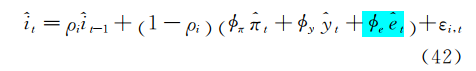# The confusion of coding the relationship between exchange rate and interest rate

Hi everyone, I am a student in DSGE.
I have a confusion of my modeling.

I build a uncovered interest rate parity:

``````de(+1) = r_h - r_f + chi_B*b;
``````

(linerized, in line 174)And I build a managed floating exchange system in a expansion taylor rule:

``````r_h = rho*r_h(-1) + (1-rho)*(phi_r*pi_h + phi_rer*de) + e_r_h;
``````

( ‘phi_rer’ is the parameter of exchange rate, and ‘de’ means ‘e/e(-1)’ , in line 163)
like this:Is it repetitive modeling? In other words, does this result in a single mechanism between interest rate and exchange rate being modeled repeatedly?

Thank you very much!

Here is the code:
nk2c0104.mod (8.0 KB)

You did not explain what `b` is? Is it foreign debt? That being said, I don’t see anything problematic here. The Taylor rule specifies a policy rule, which is independent from the rest of the model.